Next: 2.5 Minimum Tunnel Resistance Up: 2.4 Tunneling Previous: 2.4.1 Transmission Probability

## 2.4.2 Tunnel Rate

The tunnel rate from an initial state i to a final state f, considering the  change in free energy and using   Fermi's golden rule (Appendix E), is expressed as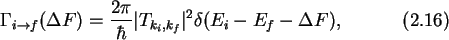where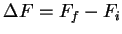is the difference in final and initial free energy. Hence a transition to a state with lower free energy produces a negative change in free energy. The difference of initial energy Ei and final energy Ef of the tunneling electron has to account for the change in free energy its transition causes. The total tunnel rate from occupied states on one side of the   barrier to unoccupied states on the other side of the barrier is given by the expression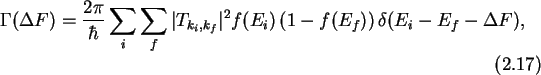where f(E) is the  Fermi-Dirac distribution, or  Fermi function which gives the  occupation probability of  energy levels. (1-f(E)) is therefore the probability of finding an empty state.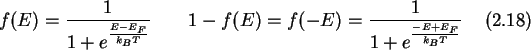For a typical  metal tunnel junction, the barrier consists of a thin oxide with a relatively high barrier height (see Fig. 2.5).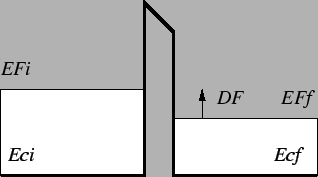It is usually a reasonable approximation for such cases to neglect the variation of the tunnel transmission coefficient with energy and momentum. The transmission probability |T|2 can then be treated as a constant which may be taken outside of the summation of (2.17).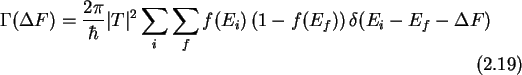Using the density of states D(E), the number of electron states in a small energy interval dE is given by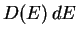. This can be used to convert the sums over momentum in (2.19) to integrals over energy.where Ec,i is the  conduction band edge of the side where the electron resides initially, Ec,f is the conduction band edge of the side the electron is tunneling to, the final side, Di(E) is the  density of states on the initial side and Df(E) is the density of states of the final side of the potential barrier. The product of the two Fermi functions defines an almost rectangular window around the Fermi energies of initial and final side (see Fig. 2.6).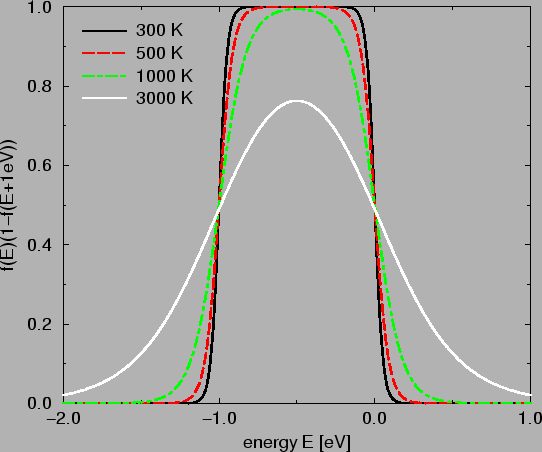Since the main contribution of the integral comes from this narrow window, the densities of states appearing in the integral may be taken constant and moved in front of the integral as well. The delta function reduces one of the integrations such that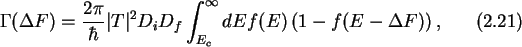where the lower limit of the integration is the higher of the two conduction band minima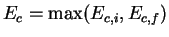. Neglecting charging effects, a tunnel junction has an Ohmic     IV-characteristic. Which means that the current through the junction is proportional to the applied bias voltage across the junction. Therefore the phenomenological quantity  tunnel resistance' can be introduced , I=V/RT. The tunnel resistance incorporates the transmission probability and the density of states.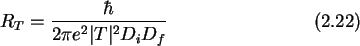Inserting (2.22) in (2.21) and using the properties of the Fermi function (see Appendix D, especially (D.5)) one gets the main result of the  orthodox' single-electron tunnel theory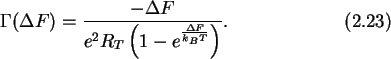For zero temperature this reduces toNext: 2.5 Minimum Tunnel Resistance Up: 2.4 Tunneling Previous: 2.4.1 Transmission Probability

Christoph Wasshuber